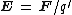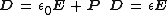# electric field

(redirected from Electric field intensity)
Also found in: Dictionary, Thesaurus, Medical.
Related to Electric field intensity: electric potential

## electric field

a field of force surrounding a charged particle within which another charged particle experiences a force
Collins Discovery Encyclopedia, 1st edition © HarperCollins Publishers 2005

## Electric field

A condition in space in the vicinity of an electrically charged body such that the forces due to the charge are detectable. An electric field (or electrostatic field) exists in a region if an electric charge at rest in the region experiences a force of electrical origin. Since an electric charge experiences a force if it is in the vicinity of a charged body, there is an electric field surrounding any charged body.

The electric field intensity (or field strength) E at a point in an electric field has a magnitude given by the quotient obtained when the force acting on a test charge q placed at that point is divided by the magnitude of the test charge q. Thus, it is force per unit charge. A test charge q is one whose magnitude is small enough so it does not alter the field in which it is placed. The direction of E at the point is the direction of the force F on a positive test charge placed at the point. Thus, E is a vector point function, since it has a definite magnitude and direction at every point in the field, and its defining equation is Eq. (1).

(1)Electric flux density or electric displacement D in a dielectric (insulating) material is related to E by either of the equivalent equations shown as Eqs. (2),
(2)where P is the polarization of the medium, and ε is the permittivity of the dielectric which is related to ε 0, by the equation ε = k ε 0, k being the relative dielectric constant of the dielectric. In empty space, D = ε 0 E .

In addition to electrostatic fields produced by separations of electric charges, an electric field is also produced by a changing magnetic field. See Electric charge, Electromagnetic induction, Potentials

McGraw-Hill Concise Encyclopedia of Physics. © 2002 by The McGraw-Hill Companies, Inc.
The following article is from The Great Soviet Encyclopedia (1979). It might be outdated or ideologically biased.

## Electric Field

a particular manifestation, along with the magnetic field, of an electromagnetic field that determines the effects upon an electric charge of a force that is independent of the velocity of the charge (see).

The concept of electric field was introduced into science by M. Faraday in the 1830’s. According to Faraday, each stationary charge creates an electric field in the surrounding space. The field of one charge acts upon another charge and vice versa, and an interaction between charges occurs (concept of short-range action). The principal quantitative characteristic of an electric field is its intensity E, defined as the ratio of the force F acting upon the charge to the magnitude of the charge q:E = F/q (seeFIELD INTENSITY, ELECTRIC). The electric field in a medium is characterized not only by its intensity but also by the electric induction vector (seeINDUCTION, ELECTRICAL AND MAGNETIC). The distribution of an electric field in space is visually represented by the intensity lines of force (seeLINES OF FORCE). The lines of force of a potential electric field that is created by electric charges originate on positive charges and terminate on negative charges. The lines of force of a rotational electric field, that is, an electric field created by a changing magnetic field, are closed curves.

The intensity of an electric field satisfies the principle of superposition, according to which for a given point in space, the intensity of the field E, created by several charges, is equal to the sum of the field intensities (E1, E2, E3, . . . .) of the individual charges: E = E1 + E2 + E3 + . . . . The superposition of fields follows from the linearity of Maxwell’s equations (seeMAXWELL’S EQUATIONS).

### REFERENCES

Tamm I. E. Osnovy teorii elektrichestva, 9th ed. Moscow, 1976. Chapters 1 and 6.
Kalashnikov S. G. Elektrichestvo, 4th ed. Moscow, 1977. (Obshchii kura fiziki.) Chapters 2 and 13.

G. IA. MIAKISHEV

## electric field

[i¦lek·trik ′fēld]
(electricity)
One of the fundamental fields in nature, causing a charged body to be attracted to or repelled by other charged bodies; associated with an electromagnetic wave or a changing magnetic field.
Specifically, the electric force per unit test charge.
McGraw-Hill Dictionary of Scientific & Technical Terms, 6E, Copyright © 2003 by The McGraw-Hill Companies, Inc.
References in periodicals archive ?
Caption: Figure 6: (a, b) The copolarized and cross-polarized electric field intensity of the transmitted waves in the x-o-z plane at 0.68 THz, respectively.
In all cases, the electric field intensity of the incident wave was 1 Volt/m.
Caption: Figure 11: Electric field intensity cloud chart on the surface of workpiece in the first laser pulse interval.
Similarly the electric field intensity and the electric flux density are related by
Electric field intensity signal 0[degrees] 45[degrees] 90[degrees] Antenna 1 0.9896 0.9705 0.9103 Antenna 2 0.9731 0.9626 0.8898 Antenna 3 0.9709 0.9609 0.9042 Antenna 4 0.9719 0.9624 0.9056 Electric field intensity Receiving signal antenna signal 135[degrees] 180[degrees] Face-to-face Antenna 1 0.9611 0.9881 0.9541 Antenna 2 0.9537 0.9758 0.9265 Antenna 3 0.9543 0.9740 0.9195 Antenna 4 0.9531 0.9753 0.9224 Table 2.
--the electric field intensity and the losses in the dielectric depend on temperature and humidity;
Using the hypotheses that describe the behavior of the moist air and the theory of the phase change, the authors deduce the two following expressions which relate the saturation pressure of the water vapor and the frost crystal critical radius of nucleation with the electric field intensity:
The resultant electric field intensity at a point 'P' at the inner surface of the outer Enclosure is given by 
A comparison between Figures 4 and 5 shows that, overall, the circular electrodes cause less intense particle velocities in the bulk of the fluid (reaching a maximum of 1.3 mm/s at the electrode edges), owing to the larger separation between neighbouring electrodes, resulting in weaker temperature (thermal increase due to ohmic heating ~ 0.7 K) and electric field intensity gradients.
Electric Field Intensity, E, is complex because the frequencies in a particular section of the earth at any time are a function of the magnetosphere, atmospherics and man-made radio and microwaves.
Pore formation is reversible or irreversible, depending on the electric field intensity, the pulse duration and the number of pulses applied.
The near-field of a dipole antenna has a distribution of electric field intensity that is very different from that given in Equation 1 for the far-field.

Site: Follow: Share:
Open / Close